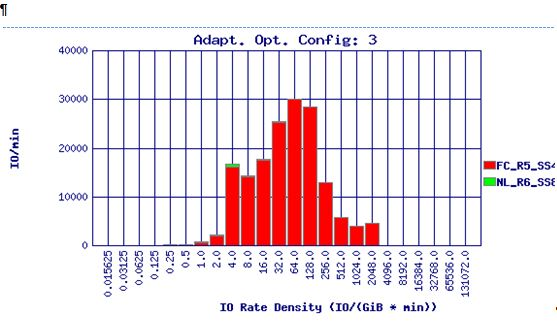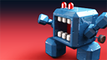HPE 3PAR StoreServ Storage

# Can anybody explain the Adaptive Optimization concept "IO Rate Density (IO/GiB * min))" to me?## Can anybody explain the Adaptive Optimization concept "IO Rate Density (IO/GiB * min))" to me?Do you see the x-axis, in the chart?  What does it mean exactly?  As near as I can tell it means the number of I/Os divided by total ( GB accessed * the number of minutes over which the IOs occurred).  So, if you had 500 Gb of space and it got 2000 IOs in 2 minutes, then that would be:

2000/(500 * 2) = 2

which would be left of center on chart.  Now 2000 IOs in 2 mins is 16 IOs/sec, which is kind of slow.

In fact, one of our HP guys told us to use this guideline:

1.  values to the left of the chart are slower, values to the right of the chart are faster.

2.  In fact, the "breaks" on the X-axis are left of 75 should be NL; 75-256 are FC; and above 256 should be SSD.

So, what does it mean exactly?

Stuart

P.S.This thread has been moved from Disk >Disk Array to Storage Area Networks (SAN) (Enterprise)- HP Forums ModeratorAcclaimed Contributor

## Re: Can anybody explain the Adaptive Optimization concept "IO Rate Density (IO/GiB * min))"

Here are some details I got from an expert:

IO Rate Density is a measure of how busy a region is.  It is calculated as illustrated in the following examples:

Consider a measurement interval of 60 minutes.  If a data region of 128 MiB (or 0.125 GiB) is accessed (reads + writes) 256 times during the 60 minutes, then the access rate density for the region is
256 accesses /( 60 minutes* 0.125 GiB ) = 34.13 accesses/GiB*min

Over the same interval (60 minutes) a meta-data region of 32 MiB (0.03125 GiB) is accessed 3090 times, the access rate density is
3090 accesses/(60 minutes * 0.03125 GiB) = 1648 accesses/GiB*min

So the meta-data region is about 48 times as busy as data region.  Hence the meta-data region would be preferred by AO over the data region for placement in a faster tier.

Consider a 15 K RPM 500 GiB drive that is capable of delivering around 240 IO/s.  If the drive were uniformly accessed (I.e.  the accesses were uniformly distributed over the entire drive), the "nominal" access rate density for the drive would be
240 IO/sec * 60 sec/minute /500 GiB = 28.8 accesses/GiB*min

In other words, if the entire drive was filled with regions with an access rate density of 28.8 access/GiB*min or higher, then the drive would be overloaded.  That obviously does not mean that we cannot place busier regions on the drive, but if we do then we would need to compensate by filling the rest of the drive with much less busy regions.

A 2TiB (2048 GiB) 7200 RPM drive that is capable of around 100 IO/s would have a "nominal" access rate density of
100 IO/sec * 60 sec/minute /1024 GiB = 5.86 accesses/GiB*min

A 200 GiB SSD drive capable of 10000 IO/sec would have a nominal access rate density of
10000 IO/sec * 60 sec/min / 200 GiB = 3000 accesses/GiB*min

So we could fill the SSD drive with regions like the meta-data region above and it would be able to handle the load.

By the way, the chart above is a histogram.  We place the regions into buckets (X-axis) based on their access rate density.
The y-axis in the chart shown is the total accesses/minute for all the regions in the given bucket.  The buckets to the right of the chart correspond to the busier regions, to the left are idler regions.

Also, a 1TiB (1024 GiB) 7200 RPM drive that is also capable of 100 IO/sec would have a "nominal" access rate density of 2x that or 11.72 access/GiB*min.  So even though the 1TiB drive is capable of the same number of IO/sec as the 2TiB drive, we can put busier regions on it because there are fewer regions.